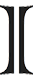# math expression on www

\large \int_{0}^{1}\frac{x^{4}\left(1-x\right)^{4}}{1+x^{2}}dx=\frac{22}{7}-\pi[/tex]

\large K = \frac{[C]^ \gamma [D]^ \delta }{[A]^ \alpha [b]^ \beta } \neq \frac{[C]^c [D]^d }{[A]^a [b]^b}

\large \sqrt{\frac{100}{27} + \frac{5\sqrt{5}}{3}} + \sqrt{\frac{100}{27} - \frac{5\sqrt{5}}{3}}

\large \int_{0}^{1}\frac{x^{4}\left(1-x\right)^{4}}{1+x^{2}}dx = \frac{22}{7}-\pirarely need to write mathematics expressions on the web, but the last time I did, it took me some times to figure out just how to do. It’d turned out to be pretty easy, there’s a free LaTeX rendering server at yourequations.com. Excellent site! Just feed it with a LaTeX expression, it would render an image for you to put on your web page. This may be the best solution for now, while waiting for an workable HTML version that supports math.

There’s also a guide to embed this feature onto Blogger blogs (and also WordPress, and some PHP forums…) Just insert your LaTeX code between the pre (or code) tags as below and the jsTeXrender JavaScript would do the rest for you. This works fine for any browser with JavaScript support (mouse over the expressions and you would see the underlying LaTeX code). You may also need this LaTeX Reference Card for a list of LaTeX’s symbols.

### Update, Otc, 1st, 2010

Due to heavy traffic, yourequations.com has ceased the service. Please see this new post on how to run a LaTeX rendering server of your own!